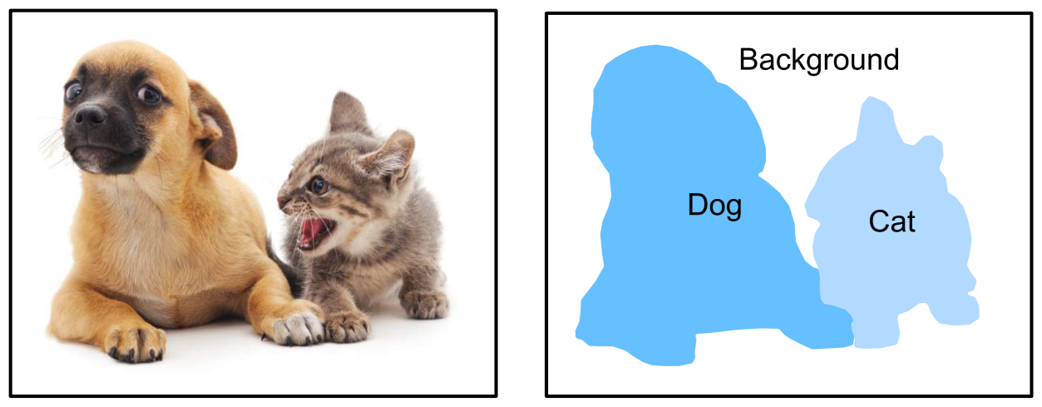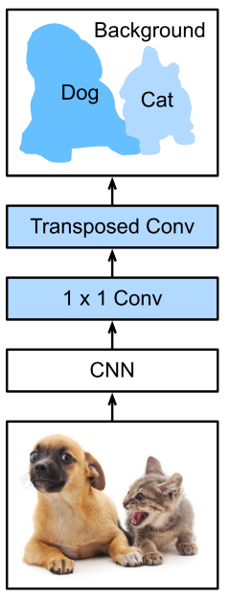Fully Convolutional Networks for Segmentation

By Prof. Seungchul Lee
http://iai.postech.ac.kr/
Industrial AI Lab at POSTECH

# 1. Segmentation¶• Segmentation task is different from classification task because it requires predicting a class for each pixel of the input image, instead of only 1 class for the whole input.
• Classification needs to understand what is in the input (namely, the context).
• However, in order to predict what is in the input for each pixel, segmentation needs to recover not only what is in the input, but also where.
• Segment images into regions with different semantic categories. These semantic regions label and predict objects at the pixel level

# 2. Fully Convolutional Networks (FCN)¶• FCN is built only from locally connected layers, such as convolution, pooling and upsampling.
• Note that no dense layer is used in this kind of architecture.
• Network can work regardless of the original image size, without requiring any fixed number of units at any stage.
• To obtain a segmentation map (output), segmentation networks usually have 2 parts

• Downsampling path: capture semantic/contextual information
• Upsampling path: recover spatial information
• The downsampling path is used to extract and interpret the context (what), while the upsampling path is used to enable precise localization (where).
• Furthermore, to fully recover the fine-grained spatial information lost in the pooling or downsampling layers, we often use skip connections.
• Given a position on the spatial dimension, the output of the channel dimension will be a category prediction of the pixel corresponding to the location.

# 3. Supervised Learning for Segmentation¶

## 3.1. Segmented (Labeled) Images¶

In :
import os
os.environ["CUDA_DEVICE_ORDER"]="PCI_BUS_ID"
os.environ["CUDA_VISIBLE_DEVICES"]="0"

In :
import numpy as np
import matplotlib.pyplot as plt
from PIL import Image

import tensorflow as tf
from keras.applications.vgg16 import VGG16

In :
train_imgs = np.load('./data_files/images_training.npy')

n_train = train_imgs.shape
n_test = test_imgs.shape

print ("The number of training images : {}, shape : {}".format(n_train, train_imgs.shape))
print ("The number of testing images : {}, shape : {}".format(n_test, test_imgs.shape))

The number of training images : 289, shape : (289, 160, 576, 3)
The number of testing images : 290, shape : (290, 160, 576, 3)

In :
idx = np.random.randint(n_train)

plt.figure(figsize = (16,14))
plt.subplot(3,1,1)
plt.imshow(train_imgs[idx])
plt.axis('off')
plt.subplot(3,1,2)
plt.imshow(train_seg[idx][:,:,0])
plt.axis('off')
plt.subplot(3,1,3)
plt.imshow(train_seg[idx][:,:,1])
plt.axis('off')
plt.show()